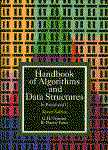## Handbook of Algorithms and Data Structures## Introduction Structure of the chapters Naming of variables Probabilities Asymptotic notation About the programming languages On the code for the algorithms Complexity measures and real timings Basic Concepts (Expand) Data structure description Algorithm descriptions Searching Algorithms (Expand) Sequential search Sorted array search Hashing Recursive structures search Multidimensional search

• Sorting Algorithms (Expand)
1. ### Techniques for sorting arrays Sorting other data structures Merging External sorting

• Selection Algorithms (Expand)
1. ### Priority queues Selection of kth element

• Arithmetic Algorithms (Expand)
1. ### Basic operations, multiplication/division Other arithmetic functions Matrix multiplication Polynomial evaluation

• Text Algorithms (Expand)
1. ### Text searching without preprocessing Searching preprocessed text Other text searching problems

• Distributions Derived from Empirical Observation
1. ### Zipf's law Bradford's law Lotka's law 80%-20% rule

• Asymptotic Expansions

• References
1. ### Textbooks Papers

• Algorithms coded in Pascal and C